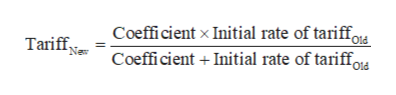# in International trade about tariff:using a simple Swiss formula to calculate the final tariff:initial rate of tariff is 120%coefficient is 8

Question
3 views

using a simple Swiss formula to calculate the final tariff:

initial rate of tariff is 120%

coefficient is 8

check_circle

Step 1

The Swiss formula for calculating final tariff rate is given below.help_outlineImage TranscriptioncloseCoeffi cient x Initial rate of tariff. Coefficient Initial rate of tariffot Tariff Old Ne Old fullscreen
Step 2

Substitute the respective values in above eq...

### Want to see the full answer?

See Solution

#### Want to see this answer and more?

Solutions are written by subject experts who are available 24/7. Questions are typically answered within 1 hour.*

See Solution
*Response times may vary by subject and question.
Tagged in